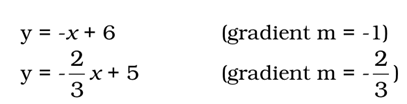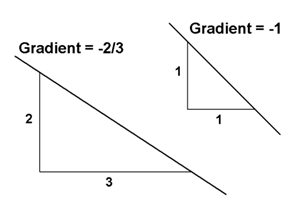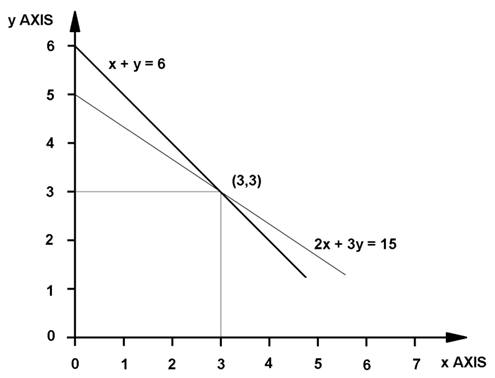# Graph

Graphs provide visual representation of data with the variation of one quantity to another. The most frequently used graph is aviation industry is a form of line graph, which uses a pair of straight lines known as axes that are drawn at right angles to each other. They are also known as Cartesian graphs or linear graphs. A pair of axes are known as rectangular or Cartesian axes. The horizontal axis is called the x-axis and the vertical axis as the y-axis. The point where these two axes meet is called as origin (0). The values on the right of the origin along the x-axis are positive and vice-versa. Likewise with the y-axis, values above the origin are positive, whilst those below are negative.

The location point on the graph is written as (x,y). The x-distance from the y-axis is called as abscissa and the y-distance from the x-axis is called as ordinate. The co-ordinates that are measure in this way are called Cartesian co-ordinates.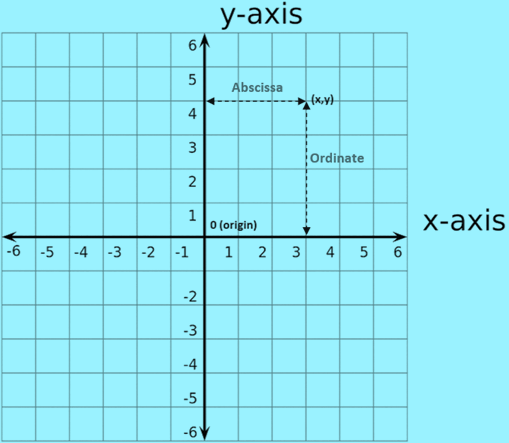### Straight Line Graph

Consider a body moves a meter for every kN force at the rate mentioned in the table below.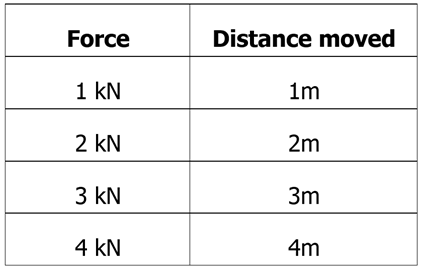The values can be represented by plotting them in a graph. It is the straight line that passes through the origin point. And the straight line shows that the output is constant.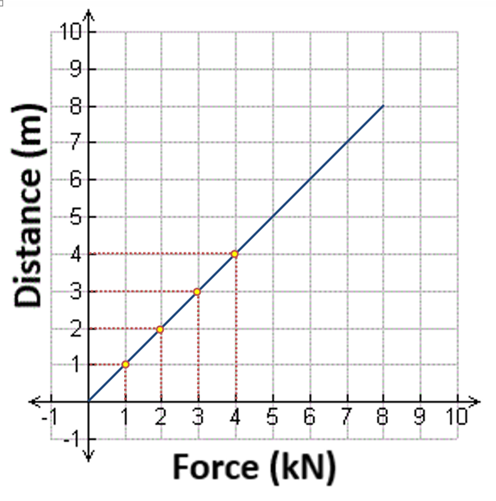### Graphing Linear Equations

Graphs are useful to find a pattern between x and y co-ordinates. The following formula shows the relationship of x and y with ‘m’ being a constant measure.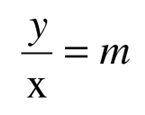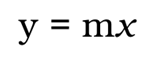When straight line graph does not pass through the origin, the relationship (y = mx) changes to (y = mx + c), where ‘c’ is a value measured on the y-axis, from the origin to where the graph cuts the y-axis (as shown below).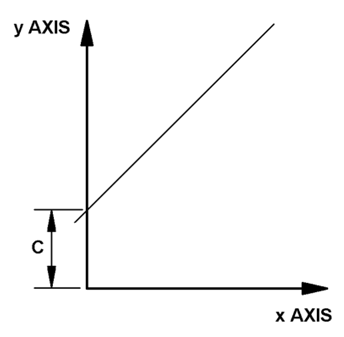Not all straight line graphs will have a positive gradient, as the one above. A straight line graph can also have a negative gradient (falling from left to right) and has a negative constant value, as shown below.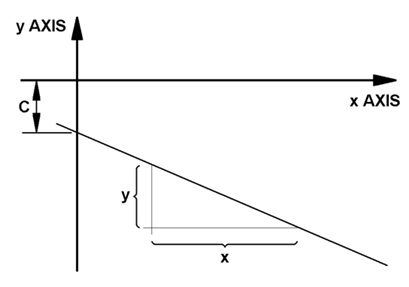Example

Sketch the graph for y – 3x = 1.

• Rearrange this into the form ‘y = mx + c’ as y = 3x + 1.
• Thus, m = 3, c = 1, y-intercept = (0,1) and slope = 3.
• Plot the points as shown in the graph below.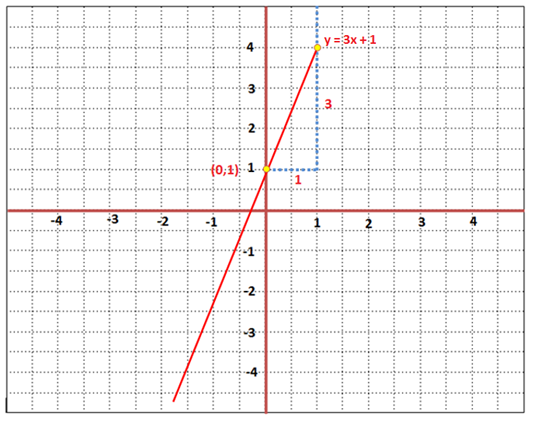Graphing Simultaneous Equations

Some forms of simultaneous equations may also be solved using this graphical method. Generally, both equations are plotted on the same graph and where the two lines intersect is the solution for x and y.

Example

Find x and y values of the equations, x + y = 6 and 2x + 3y = 15 using graph method.

Rearrange this into the form ‘y = mx + c’ and get the following equations.